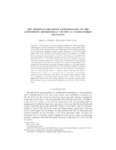## The Primitive equations approximation of the anisotropic horizontally viscous 3D Navier-Stokes equations

##### Authors
Li, Jinkai
Titi, Edrisshttps://orcid.org/0000-0002-5004-1746
Yuan, Guozhi
##### DOIArticle
##### Abstract

In this paper, we provide rigorous justification of the hydrostatic approximation and the derivation of primitive equations as the small aspect ratio limit of the incompressible three-dimensional Navier-Stokes equations in the anisotropic horizontal viscosity regime. Setting ε >0 to be the small aspect ratio of the vertical to the horizontal scales of the domain, we investigate the case when the horizontal and vertical viscosities in the incompressible three-dimensional Navier-Stokes equations are of orders O(1) and ε^α, respectively, with α>2, for which the limiting system is the primitive equations with only horizontal viscosity as ε tends to zero. In particular we show that for "well prepared" initial data the solutions of the scaled incompressible three-dimensional Navier-Stokes equations converge strongly, in any finite interval of time, to the corresponding solutions of the anisotropic primitive equations with only horizontal viscosities, as ε tends to zero, and that the convergence rate is of order O(ε^(β/2)), where β=min{α-2,2}. Note that this result is different from the case α=2 studied in [Li, J.; Titi, E.S.,The primitive equations as the small aspect ratio limit of the Navier-Stokes equations: Rigorous justification of the hydrostatic approximation, J. Math. Pures Appl., Vol. 124, (2019), 30-58], where the limiting system is the primitive equations with full viscosities and the convergence is globally in time and its rate of order O(ε).

2021-11-04
2021-10-26
##### Journal Title
Journal of Differential Equations
0022-0396
Elsevier